# RS Aggarwal Solutions for Class 8 Maths Chapter 1 - Rational Numbers Exercise 1B

RS Aggarwal Solutions for Class 8 Maths Chapter 1- Exercise 1B, Rational Numbers are provided here. Students can download from the links provided below. Solutions are solved by our experts in order to help students in learning the concepts with ease from the RS Aggarwal Solutions textbook. This ensures that the students are thorough with their basic concepts by practicing the solutions.

In Exercise 1B of RS Aggarwal Class 8 Maths, we shall study the Representation of Rational Numbers on the Real Line.

## Download PDF of RS Aggarwal Solutions for Class 8 Maths Chapter 1- Rational Numbers Exercise 1B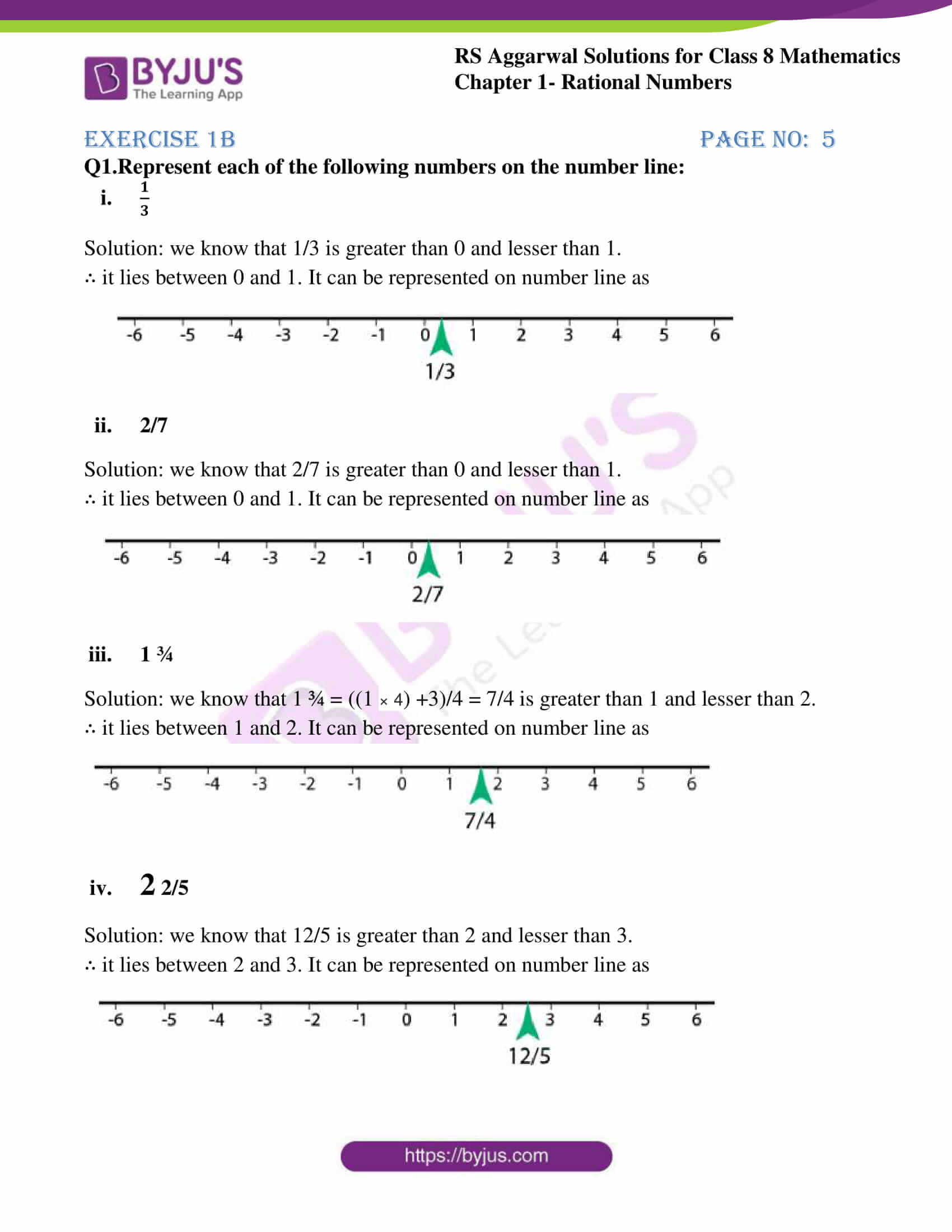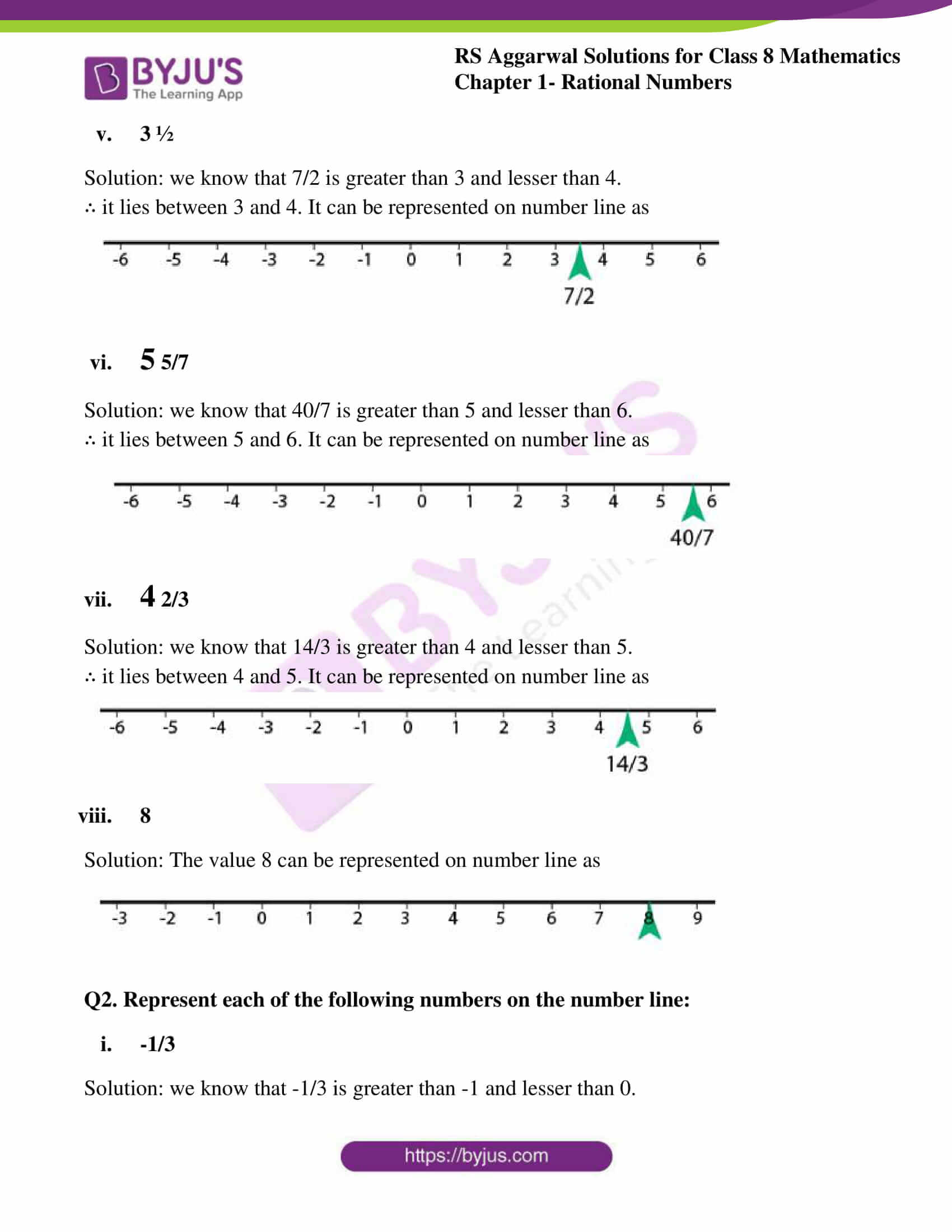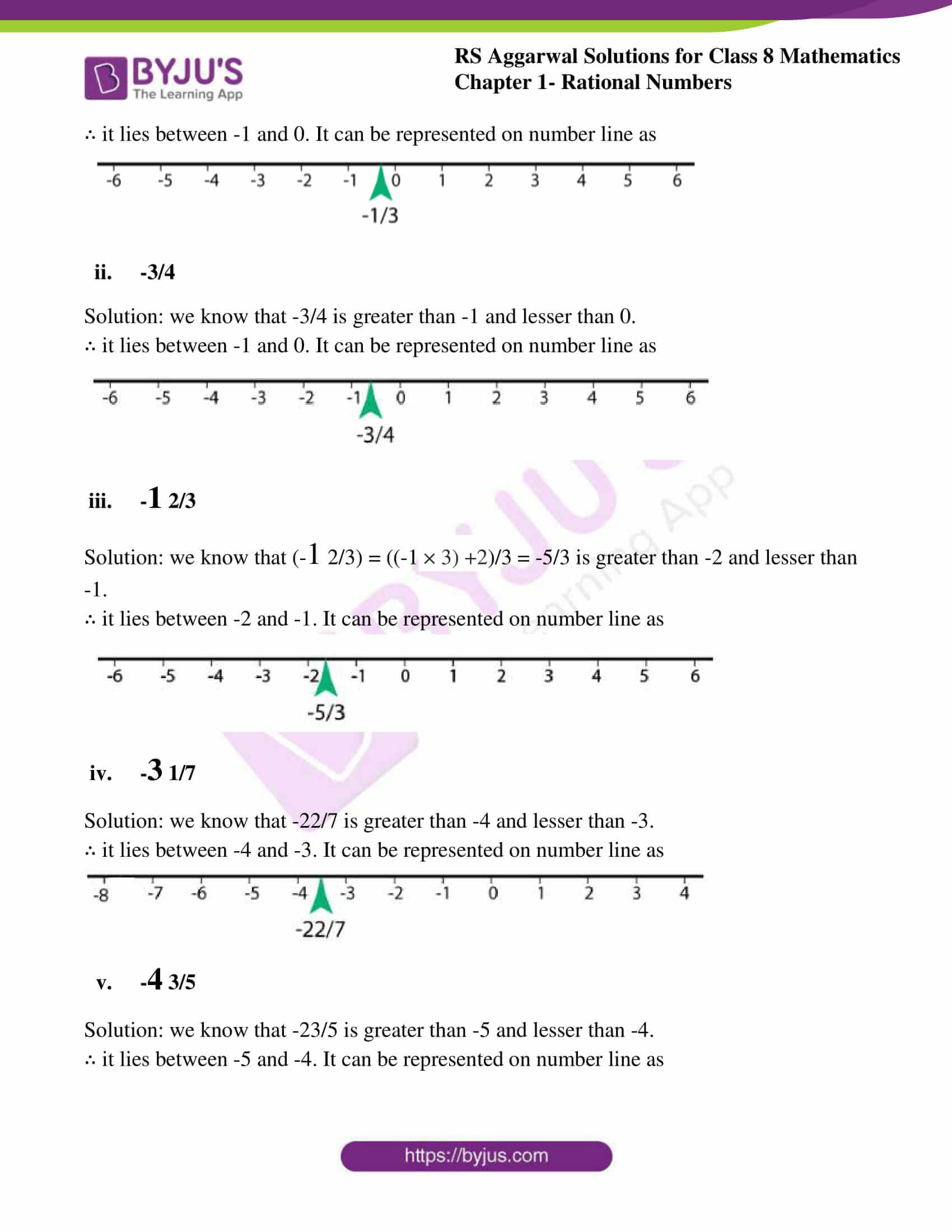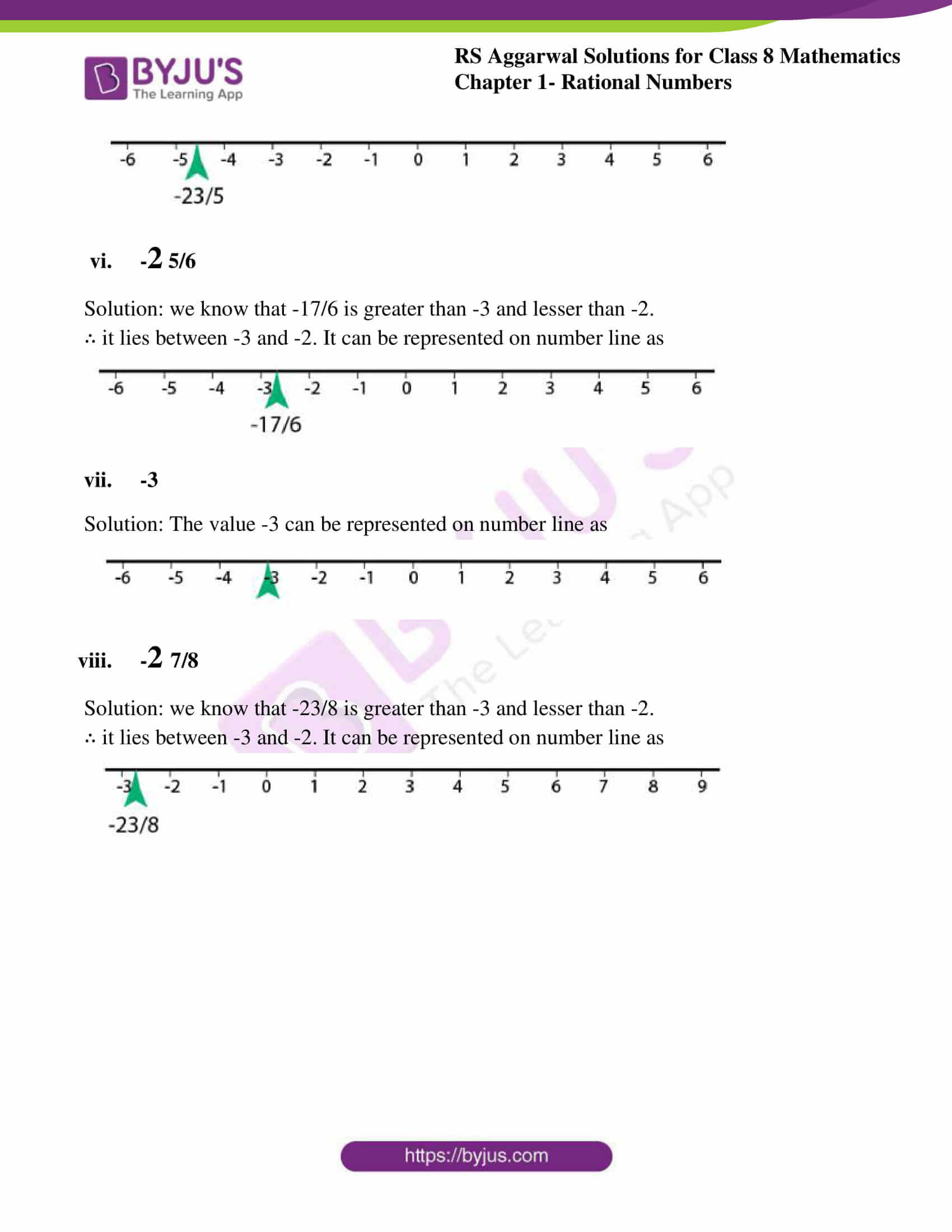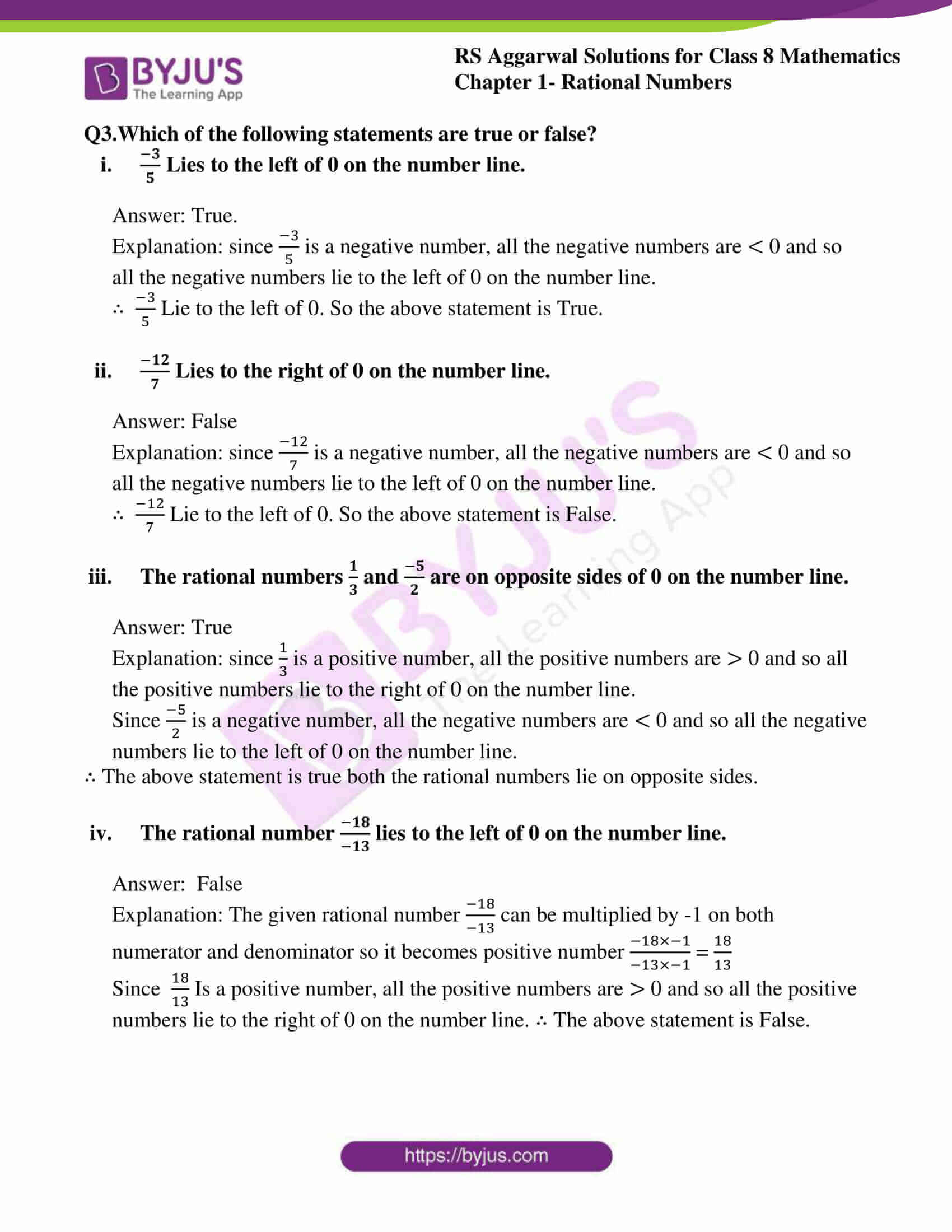### Access Answers to RS Aggarwal Solutions for Class 8 Maths Chapter 1- Rational Numbers Exercise 1B

Q1.Represent each of the following numbers on the number line: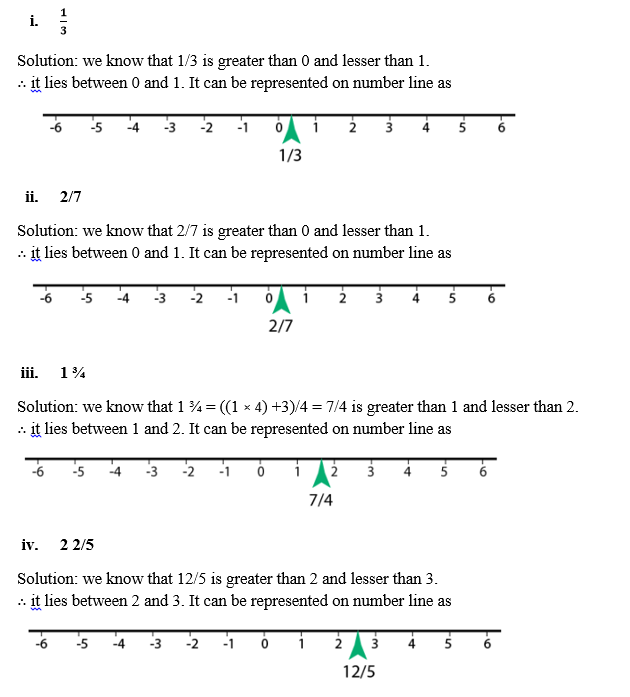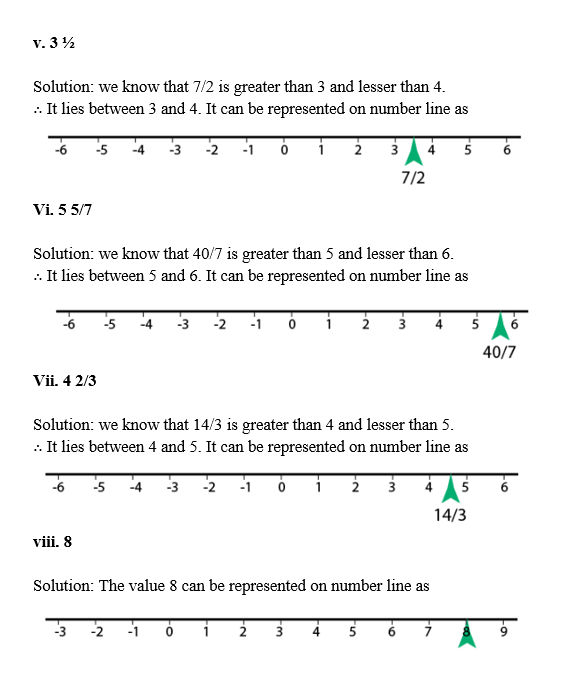Q2. Represent each of the following numbers on the number line: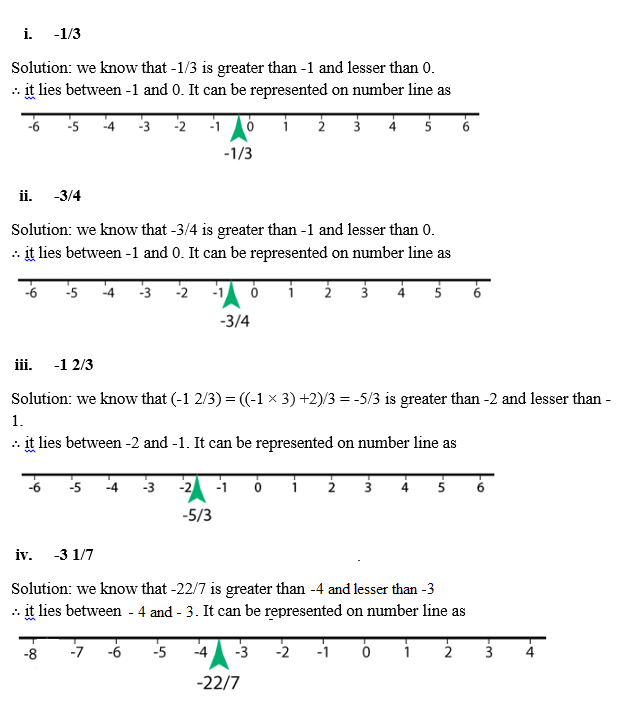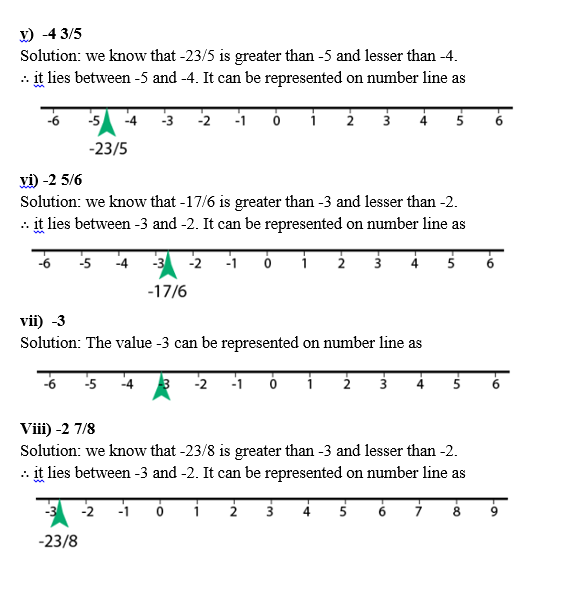Q3. Which of the following statements are true or false?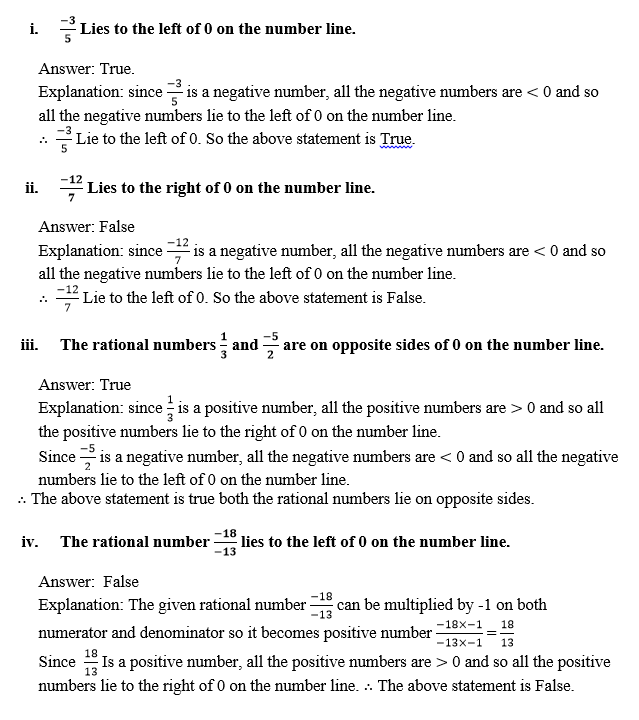### Access other Exercises of RS Aggarwal Solutions for Class 8 Maths Chapter 1- Rational Numbers

Exercise 1A Solutions 10 Questions

Exercise 1C Solutions 14 Questions

Exercise 1D Solutions 9 Questions

Exercise 1E Solutions 12 Questions

Exercise 1F Solutions 8 Questions

Exercise 1G Solutions 20 Questions

Exercise 1H Solutions 23 Questions

## RS Aggarwal Solutions for Class 8 Maths Chapter 1- Rational Numbers Exercise 1B

Exercise 1B of RS Aggarwal Class 8 Chapter 1, Rational Numbers contains the basic concepts related to Rational Numbers. This exercise mainly deals with the representation of Rational Numbers on the Real Line.

The RS Aggarwal Solutions can help the students practice and learn the fundamentals as it provides all the answers to the questions from the RS Aggarwal textbook. By practicing as many times as possible helps them in scoring high marks.